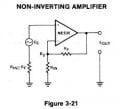# How to figure out the maximum current flowing into your op-amp

#### MikeJacobs

Joined Dec 7, 2019
221
So i am trying to figure out how to understand what the maximum current flowing into and or out of depending on construction of the non inverting and inverting terminals of an op amp

I know its some combination of input bias current with some addtion of input offset current.

Can someone explain a bit more?
Thanks

#### MrChips

Joined Oct 2, 2009
29,251
The input resistance of most opamps is so high it would be futile trying to measure the input current.

#### MikeJacobs

Joined Dec 7, 2019
221
I understand that but its not a futile effort when trying to understand the error of an op-amp
that total current multiplied by a very high input impedance gives way to significant voltage error

#### crutschow

Joined Mar 14, 2008
32,933
The voltage error is the op amp input offset voltage multiplied by the non-inverting gain of the circuit (even if it's configured as an inverting amp).
The bias voltage error is equal to the input bias current times the feedback resistance.

The op amp input bias current is unrelated to the input voltage offset.

#### MikeJacobs

Joined Dec 7, 2019
221
The voltage error is the op amp input offset voltage multiplied by the non-inverting gain of the circuit (even if it's configured as an inverting amp).
The bias voltage error is equal to the input bias current times the feedback resistance.

The op amp input bias current is unrelated to the input voltage offset.

I am completely dumbfounded as to why you would say the voltage offset has nothing to do with the input bias current.
Case in point the voltage offset error is caused by the input bias current multiplied by the input impedance.
I know you have been around a long time but are you sure you did not just mis speak there?
Thanks

#### ericgibbs

Joined Jan 29, 2010
18,064
hi Mike,
Check thru this PDF, using Search.
E

#### Attachments

• 2 MB Views: 5

#### MikeJacobs

Joined Dec 7, 2019
221
Thanks for the doc, what should i be looking for. Its 460 pages

#### ericgibbs

Joined Jan 29, 2010
18,064
hi,
For example.
E

#### Ian0

Joined Aug 7, 2020
8,425
The voltage error due to the bias current is the bias current multiplied by the resistance to ground, not by the input impedance. This voltage error is added to the offset voltage of the op-amp, and the error at the output is the sum of the two multiplied by the DC voltage gain of the circuit.
The maximum input bias current is quoted in the datasheet. Use this figure to calculate the maximum error. Measuring it for an individual op-amp and using that figure for calculations will lead to problems if the op-amp is ever replaced.

#### schmitt trigger

Joined Jul 12, 2010
702
Opamp manufacturers have already measured it for you. It is in the datasheet.
For instance the ubiquitous LM358. The information is on page 4, paragraph 3.
You may note that the units are nano amps. And the 358 is not considered a low bias current opamp.

https://www.onsemi.com/pub/Collateral/LM358-D.PDF

#### MikeJacobs

Joined Dec 7, 2019
221

What is the total current into the terminal?

Is it Input bias + Input offset?

#### crutschow

Joined Mar 14, 2008
32,933
I am completely dumbfounded as to why you would say the voltage offset has nothing to do with the input bias current.
I'm referring to the bias current at the input terminals to the op amp, not the current flowing into any of the bias resistors.
The op amp bias is basically unaffected by any other parameters.

Last edited:

#### dl324

Joined Mar 30, 2015
15,847
So i am trying to figure out how to understand what the maximum current flowing into and or out of depending on construction of the non inverting and inverting terminals of an op amp
It's in the datasheets.

Normally we don't worry about input bias currents. Normal design guidelines tell you to match the impedances on the inputs so the effects of bias currents will cancel.

This is from a 1979 Signets Analog Applications Handbook:#### MrChips

Joined Oct 2, 2009
29,251
Generally speaking, op-amps are used to amplify DC or AC.
If you are working with AC signals then you can remove the DC offset.
If you are working at 0Hz then you have at lot more to have worry about.

#### Ian0

Joined Aug 7, 2020
8,425

What is the total current into the terminal?

Is it Input bias + Input offset?
Just input bias.
(Input offset is a voltage)

#### MikeJacobs

Joined Dec 7, 2019
221
Just input bias.
(Input offset is a voltage)
However i don't believe this to be true. I have found a few things that tell me other wise. My apologies for the typo. I meant to say input offset current.

#### Ian0

Joined Aug 7, 2020
8,425
I have found a few things that tell me other wise.

#### MikeJacobs

Joined Dec 7, 2019
221